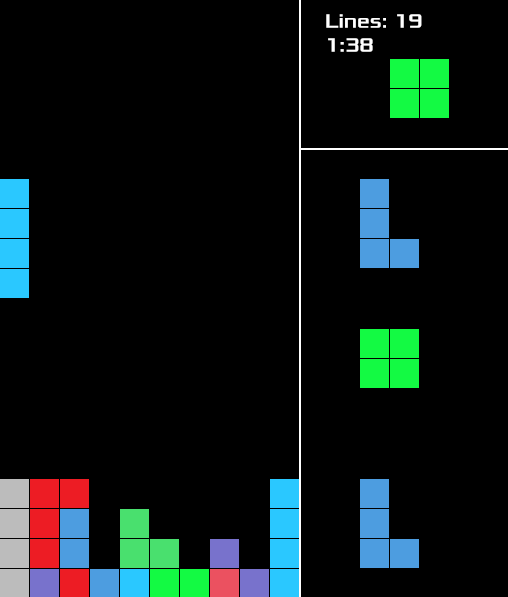I wrote tetrs (https://github.com/mkhan45/tetrs) over the summer after playing Tetris with some friends on https://jstris.jezevec10.com/. One of my goals when writing it was for it to be written mostly using functional programming. I did complete the actual Tetris part back then, but the UI was pretty bad so I never really used it. I finally got around to finishing it a few weeks ago because of coronabreak.

As usual, I wrote it in Rust using `ggez` as a game engine.The main difficulties in writing Tetris come a few main areas:

• Block collision
• Tetromino rotation
• Projection for the predicted tetromino placement at the bottom

A few months ago I read a post on r/programminghorror or somewhere similar about tetris collision detection function that was hundreds of lines long. As it turns out, Tetris collision detection is really simple if you separate tetrominoes and squares. For that reason, the logic for the board is separated into 3 structs:

• `Square` - A single square, logically just a position tuple but it also has `Rect` and `Color` fields for drawing.
• `Block` - A tetromino, but I didn't know that they were called tetrominoes at the time. This is composed of an array of 4 `Square`s, a `BlockType` enum, and an `Orientation` enum.
• `GameState` - well a `GameState` has the state of the whole game; the relevant fields are `squares`, an expandible array of squares, and `current_block`, which is a `Block`. I thought about having an array of `Option<Square>`s for `squares`, but a preallocated `Vec<Square>` is easier.

With these structs, collision is easily detected by checking if any of the `squares` in `GameState` have the same position tuple as any of the squares in `current_block`. Functional programming ideas help here. `Block` has a `translate` method. With normal OOP, you'd probably make the signature `fn translate(&mut self, x, y)`, but to preserve immutability, I used `fn translate(&self, x, y) -> Block`.

This is helpful later on in this method in `GameState`:

``````pub fn try_translate(&mut self, x: i8, y: i8) {
let translated = self.current_block.translate(x, y);
if translated.is_valid(&self.squares) {
self.current_block = translated;
}
}``````

I don't actually use `try_translate` on `current_block` because a lot more stuff has to happen if `translated` is not valid. Instead I did this:

``````// create a temporary block that is the current_block translated down by one
let translated = self.current_block.translate(0, 1);

if translated.is_valid(&self.squares) {
self.current_block = translated;
} else {
// handle everything that has to do with the current_block colliding
...
}``````

Tetromino rotation was a lot less elegant. As far as I know, it must be hardcoded. The bulk of the logic is in `Block::new()`, which just specifies an array of squares for every combination of `BlockType` and `Orientation`. I managed to make it look okay though. Here's a snippet:

``````(BlockType::T, Orientation::Up) => {
block_from_squares(blocktype, orientation,
[
(1, 0),
(0, 1), (1, 1), (2, 1)
]
)
}``````

Of course, autoformatting is turned off for that function.

Rotation is also hardcoded because blocks have to be translated a bit on rotation to remain consistent. There's some standard way to rotate blocks that I followed.

Making the projection at the bottom was surprisingly difficult. It would be easy to just test every downward translation of the current block, but that would be pretty inefficient. Instead, I tried to find the max drop distance for each square in the block. At first I filtered it to only include the squares at the bottom of the tetromino, but there's only 4 anyway so that actually makes it slower.

This function finds the max distance that a tetromino can be translated down before hitting something:

``````pub fn max_drop(&self, board: &[Square]) -> i8 {
self.squares.iter().fold(Y_SQUARES + 5, |max_dist, square| {
let square_max = square.max_y_translate(board);
if square_max < max_dist {
square_max
} else {
max_dist
}
}) - 1
}``````

`Y_SQUARES + 5` is the max distance a square could be dropped from the time it was spawned.

`square.max_y_translate` is shown here:

``````pub fn max_y_translate(&self, board: &[Square]) -> i8 {
// starts by filtering the board to only squares on the same x axis, and then
// looks down
let max_square = board
.iter()
.filter(|square| square.pos.0 == self.pos.0 && square.pos.1 >= self.pos.1)
.fold(Square::bottom(self.pos.0), |max_square, current_square| {
if current_square.pos.1 <= max_square.pos.1 {
*current_square
} else {
max_square
}
});
max_square.pos.1 - self.pos.1
}``````

Overall, I'm pretty happy with how it turned out. I was able to go back to it after six or so months and add a main menu and fix some bugs without too much difficulty, and I don't think there's too much inefficiency anywhere.